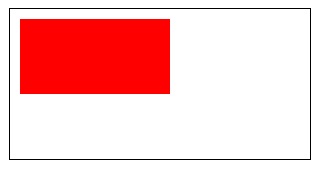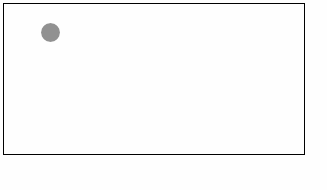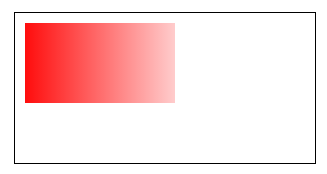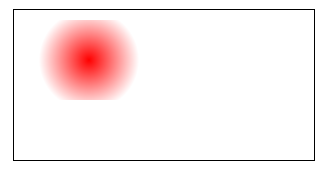## # Canvas

All the drawings in canvas must be completed with JavaScript:

WXML: (Unless otherwise specified, WXML is used as a template in the following example)

``````<canvas canvas-id="myCanvas" style="border: 1px solid;"/>
``````

JS: (JS is placed in onLoad in the following example)

``````const ctx = wx.createCanvasContext('myCanvas')
ctx.setFillStyle('red')
ctx.fillRect(10, 10, 150, 75)
ctx.draw()
``````

Step 1: Create a Canvas drawing context

First, we need to create a Canvas drawing context CanvasContext.

CanvasContext is an object built in the Mini Program, featuring several drawing methods:

``````const ctx = wx.createCanvasContext('myCanvas')
``````

Step 2: Use the Canvas drawing context to describe the drawing

Next, we will describe what content to draw on the Canvas.

Set the fill color of the drawing context to red:

``````ctx.setFillStyle('red')
``````

Draw a rectangle using the `fillRect(x, y, width, height)` method, and fill it with red as just set:

``````ctx.fillRect(10, 10, 150, 75)
``````

Step 3: Draw

Tell the canvas component to draw the content you just described:

``````ctx.draw()
``````

Result:## # Coordinate System

Canvas is a two-dimensional grid. The coordinates of the upper left corner are `(0, 0)`.

In the previous section, we used the method `fillRect(0, 0, 150, 75)`.

This indicates that, starting from the upper left corner `(0, 0)`, draw a `150 x 75` px rectangle.

Code sample

We can add events in canvas to observe its coordinate system.

``````<canvas canvas-id="myCanvas"
style="margin: 5px; border:1px solid #d3d3d3;"
bindtouchstart="start"
bindtouchmove="move"
bindtouchend="end"/>

<view hidden="{{hidden}}">
Coordinates: ({{x}}, {{y}})
</view>
``````
``````Page({
data: {
x: 0,
y: 0,
hidden: true
},
start (e) {
this.setData({
hidden: false,
x: e.touches.x,
y: e.touches.y
})
},
move (e) {
this.setData({
x: e.touches.x,
y: e.touches.y
})
},
end (e) {
this.setData({
hidden: true
})
}
})
``````

When you put your finger on the canvas, the coordinates of the point you touch are displayed at the bottom of the page:A gradient can be used to fill a rectangle, circle, line, text, etc. The fill color is not always the same.

Two color gradient methods are available:

The `addColorStop(position, color)` method is used to specify the position and color of a color gradient point. The position must be between 0 and 1.

You can use the `setFillStyle` and `setStrokeStyle` methods to set the gradient and then describe the drawing.

Use `createLinearGradient()`

``````const ctx = wx.createCanvasContext('myCanvas')

const grd = ctx.createLinearGradient(0, 0, 200, 0)

ctx.setFillStyle(grd)
ctx.fillRect(10, 10, 150, 80)
ctx.draw()
``````Use `createCircularGradient()`

``````const ctx = wx.createCanvasContext('myCanvas')